# Visualizing group structure with colored addition/multiplication tables

When working with finite fields, if the number of elements is a prime power$p^m$ with m > 1, you can represent the elements as polynomials with degree m-1 and do the field addition and multiplication modulo a irreducible polynomial with degree m.

The field GF(5) is composed by the numbers 0 to 4. We don’t need to represent its elements as polynomials since m=1. Addition is done modulo 5 and multiplication also modulo 5. So 2 + 3 = 0; 4 * 2 = 3; and so on. This is the addition table for GF(5):

The rows, top down, represent 0 to 4. The columns, right to left, represent 0 to 4. Each square is the result of the addition of the respective numbers in the row / column it belongs to. Black is 0, purple is 1, red is 2, orange is 3, yellow is 4.

In the field GF(25) = GF(5²), as I said, you represent each element as a polynomial. So we have 25 elements: 0 to 4; x, x+ 1, …, x + 4; 2x, 2x + 1, …; 3x, 3x + 1, …; 4x, 4x + 1, …, 4x + 4.

In order to add two elements, add them as you would add two polynomials, but remember that the coefficients are in GF(5); for example, in GF(5²), we have (3x + 2) + (4x + 4) = (2x + 1). In order to multiply two elements, multiply them as usual but then take the result modulo an irreducible polynomial. So, with GF(5²) modulo$x^2 + 4x + 2$, you have (2x + 5) * (3x + 4) = (4x + 3).

I always wondered what would happen when you changed the modulus. Obviously the group “changes”, but in order to actually see it, I’ve built the multiplication table for GF(5²) modulus$x^2 + 4x + 2$ and$x^2 + 3x + 3$: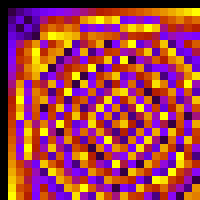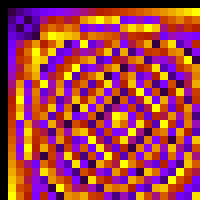Yep, they’re different 😀

Of course, both are isomorphic, so you’re free to pick your favorite modulus.

### Multiplicative group of integers modulo n vs group of points in a elliptic curve

Then I got curious: how would the multiplication table of integers modulo n look like? This group is the group used in many cryptographic schemes, like RSA. This is the multiplication table for integers modulo 509: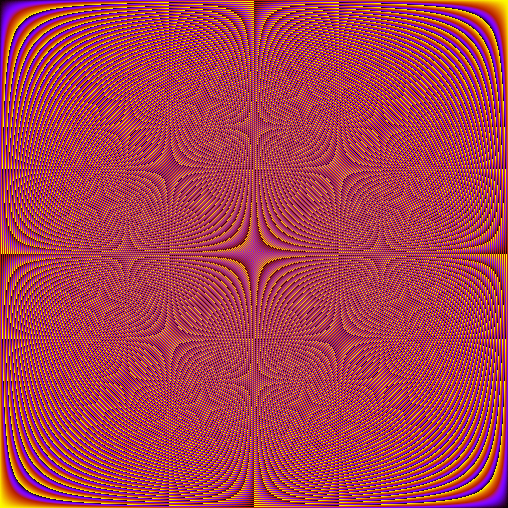Pretty (and trippy)!

What about the group of points on a elliptic curve over a finite field, which is also a group used in cryptographic schemes? This is the additive table for the points on$y^2 = x^3 + 4x + 1$ over GF(503):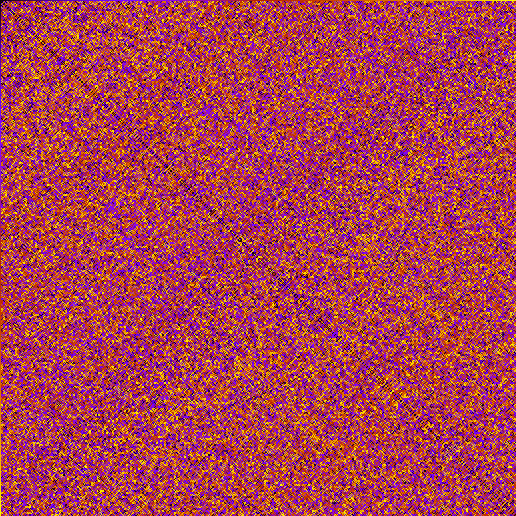The difference between them is striking; the elliptic group seems almost random. This is (intuitively speaking! I’m not being formal here) the reason why this group is used in cryptography in the first place: since the group structure is more “messed up”, you can get away with using groups of much smaller size (no smaller than$2^{160}$ elements) than with multiplicative groups of integers modulo n (no smaller than$2^{1024}$ elements). This is not set in stone though; maybe someday someone will come up with a better method to crack this seemingly random structure (for now the best method to solve the discrete log problem for elliptic groups is exponential, while the best method for integers modulo n is sub-exponential).

It’s worth mentioning that even this elliptic group is not that extraordinary: it is isomorphic to the very simple additive group of integers modulo 506: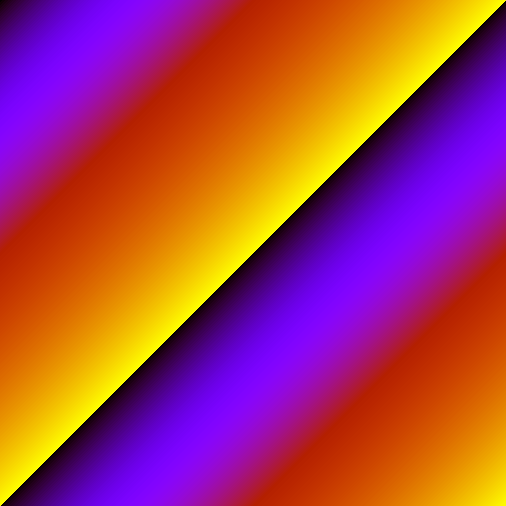The big problem is to find the isomorphism! You can see this better with a small example. This is the elliptic group of$y^2 = x^3 + 3x + 2$ over GF(5) (left) which is isomorphic to the additive group modulo 5 (right):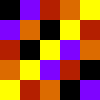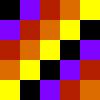(OK, it’s not that easy to see)

### Software

To generate those images, I’ve used Python with PIL and Sage. Sage aims to be a open source replacement for (expensive) software like Magma, Maple, Mathematica and Matlab. Since I’ve never used those, I can’t really say how it is going in its mission, but it’s really awesome. If you’re a Windows user you’ll probably be scared by the fact that the Windows version of Sage is actually an entire Linux virtual machine! They’re working to port it natively, but even until then, it’s worth it (and you’ll have an excuse to try Linux 😉 )

### Update

Someone asked for the source code used to generate those. It’s ugly (I’ve added comments at least) but you can download it here: the sage script and the python script. In the sage script, uncomment the lines representing what you want to plot, then run `./sage gentable.sage` (or whichever path to were sage is). It will generate a data.txt in the same folder. Now run `python plottable.py img.png` to plot it on the img.png file (or omit it to show on the screen). You’ll need to have PIL installed.

If you don’t want to plot fancy stuff as elliptic groups, you can easily transform the gentable.sage into a normal Python script and write the addition/multiplication yourself (like a + b % n). Have fun, and feel free to ask anything.

1.Alan Crowe says:

Wow! I gasped aloud at the sight of y^2 = x^3 + 4x + 1 over GF(503). I’ve dumped random bits into images to play with smoothing algorithms and they look just like that before you smooth them. (The gray scale ones turn into slate paving slabs when you smooth them enough, I’ve forgotten what happened to the colour ones.)

2.andrewl says:

“Then I got curious: how would the multiplication table of integers modulo n look like? This group is the group used in many cryptographic schemes, like RSA. This is the multiplication table for integers modulo 509:”

Well where is the n? 509 is prime!

If you draw a picture of the group Z_n*, it would be very cool if you could draw by its side Z_p* and Z_q* and then use the same colors in Z_n* somehow so that we could see the “direct product” of the two groups.

3.Conrado says:

@andrewl:

You’re right, I’ve messed up 🙂 I should’ve mentioned something like DSA instead.

Your idea is neat, I’ll try it later and post it. Thanks for reading!

4.Steve Souza says:

Interesting. I created a simple java program a while back that allows you to put in any number you want to use as the modulo. The results are drastically different depending on what you pick. Also it generates some images using a tangent function. The code and a couple examples of what is generated are attached. The modulo images seem to have been removed though…

http://www.coderanch.com/forums/forums/posts/watch/0/35507

5.www.wakonda.net says:

continuously i used to read smaller articles which also clear their motive,
and that is also happening with this paragraph which I am reading now.

6.telha curitiba says:

Meu membros da família cada vez dizem que eu sou matando meu tempo aqui no líquido , no entanto eu
sei que estou recebendo conhecimento o tempo todo lendo tais fastidioso
postos .